# 7 Segment LED display driver with Ada on STM32F4 Discovery

A simple display driver for 7 Segment LED displays of any arbitary number of digits, controlled directly using GPIOs. programmed in Ada.

IntermediateFull instructions provided10 hours2,989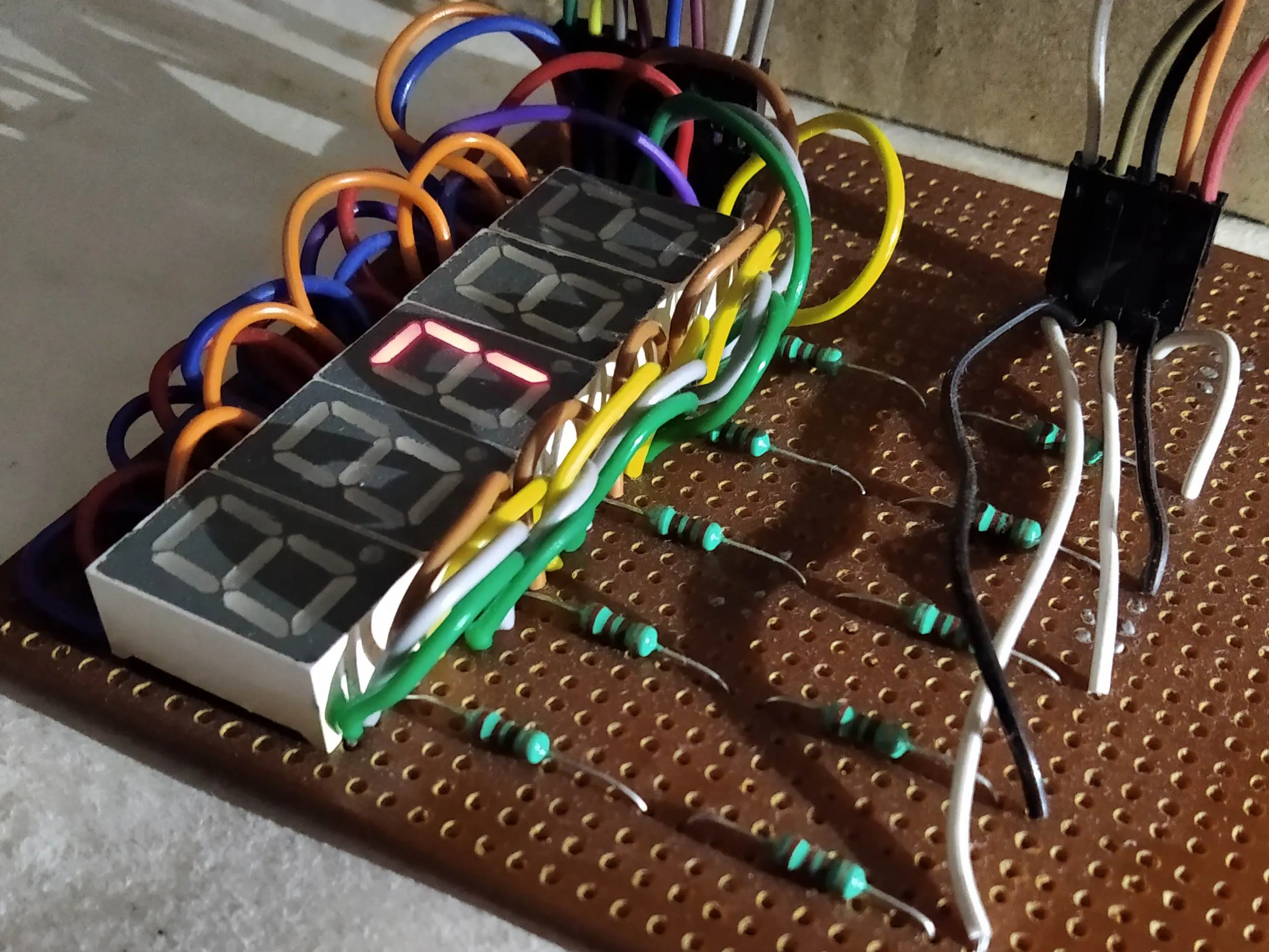## Things used in this project

### Hardware components

 STMicroelectronics STM32F407 Discovery
×1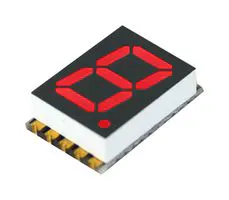7 Segment LED Display, Red
×5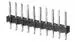Male Header 40 Position 1 Row (0.1")
×1

### Software apps and online services### Hand tools and fabrication machinesSoldering iron (generic)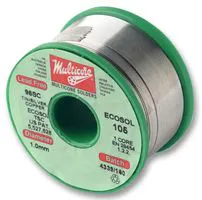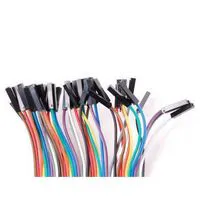Premium Female/Female Jumper Wires, 40 x 6"

## Schematics

### seven segement display schematic

circuit diagram of seven segment units connected together to create a display.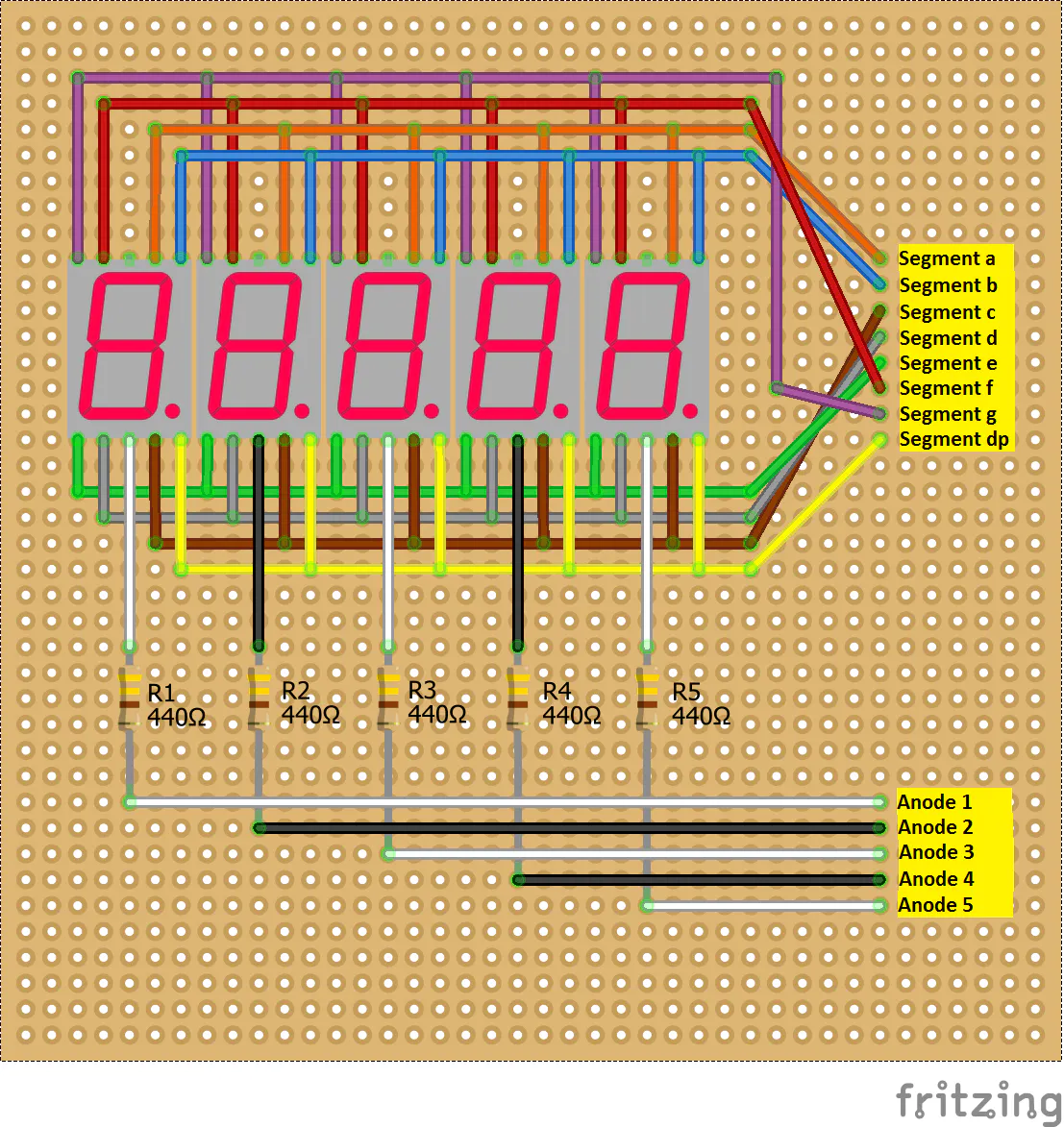## Code

The GNAT Project file
```with "../../../boards/stm32f407_discovery/stm32f407_discovery_full.gpr";

for Target use "arm-eabi";
for Source_Dirs use ("src");
for Object_Dir use "obj";
for Create_Missing_Dirs use "True";

package Compiler renames STM32F407_Discovery_Full.Compiler;

```

```with display_value;              pragma Unreferenced (display_value);
--  These packages contains the task that actually controls the app so
--  although it is not referenced directly in the main procedure, we need it
--  in the closure of the context clauses so that it will be included in the
--  executable.

with Last_Chance_Handler;  pragma Unreferenced (Last_Chance_Handler);
--  The "last chance handler" is the user-defined routine that is called when
--  an exception is propagated. We need it in the executable, therefore it
--  must be somewhere in the closure of the context clauses.

with System;

procedure seven_segs_test is
pragma Priority (System.Priority'First);
begin
loop
null;
end loop;
end seven_segs_test;
```

```package body display_value is

begin

num_tx := -5.2;

infinite_loop :
loop

num_tx := num_tx + 0.1;

store_data.put_data (num_tx);

delay until Clock + Milliseconds (1000);

end loop infinite_loop;

end send_data;

end display_value;
```

```with display_config;    use display_config;
with display_driver;    use display_driver;
with display_po; use display_po;
with System;

pragma Elaborate_Body;

temp_integer : Constant Integer := 5;

my_display : constant display_parameters := (decimal_point => real_no, signed_number => signed_no, Fore_digits => 3, Aft_digits => 1);

string_representation_data : String( 1 .. output_string_length(my_display) ) := (Others => ' ');

raw_data : Float := 0.0;

procedure My_Delay (Milliz : Natural);

pragma Priority (System.Priority(15));
end display_data;

```

```package body display_task is

procedure My_Delay (Milliz : Natural) is
begin
delay until Clock + Milliseconds (Milliz);
end My_Delay;

delay_digit_show : constant Natural := 5;
--not required.
--delay_digit_sweep : constant Natural := 1;
begin

beyond_infinity:
loop

-- read the number to be displayed.
raw_data := store_data.get_data;

-- convert it to the string for ease of manipulation further.
string_representation_data := to_display_string(raw_data, my_display);

-- generate a frame containing boolean status for each segment for each digit.
generate_frame(string_representation_data);

-- now display on the seven segment display.
sweep_digits :
for i in digit_anodes'Range loop

sweep_segments :
for j in seven_segs'Range loop
if (current_frame(i, j) = True) then
Segment_On(seven_segs(j));
else
Segment_Off(seven_segs(j));
end if;

end loop sweep_segments;

Digit_On(digit_anodes(i));
My_Delay(delay_digit_show);
Digit_Off(digit_anodes(i));

end loop sweep_digits;

end loop beyond_infinity;

end display_data;

procedure display_init_state is
begin
all_digits_off :
for i in digit_anodes'Range loop
Digit_Off(digit_anodes(i));
end loop all_digits_off;

all_segments_off :
for i in seven_segs'Range loop
Segment_Off(seven_segs(i));
end loop all_segments_off;

end display_init_state;

procedure Initialize_devices is
begin
Initialize_segments;
Initialize_digits;
display_init_state;
end Initialize_devices;

begin

Initialize_devices;

```

```with STM32.Device;  use STM32.Device;
with STM32.GPIO;    use STM32.GPIO;
with Ravenscar_Time;

package display_config is

pragma Elaborate_Body;

-- Hardware mapping

subtype segment_pin is GPIO_Point;
subtype common_pin is GPIO_Point;

Segment_a : segment_pin renames PA0;
Segment_b : segment_pin renames PA1;
Segment_c : segment_pin renames PA2;
Segment_d : segment_pin renames PA3;
Segment_e : segment_pin renames PA4;
Segment_f : segment_pin renames PA5;
-- Not using the PA6 as, experienced some issues with this pin when used as GPIO.
--Segment_g : segment_pin renames PA6;
Segment_g : segment_pin renames PC5;
Segment_dp : segment_pin renames PA7;

anode_1 : common_pin renames PE7;
anode_2 : common_pin renames PE8;
anode_3 : common_pin renames PE9;
anode_4 : common_pin renames PE10;
anode_5 : common_pin renames PE11;

seven_segs : GPIO_Points := (Segment_a, Segment_b, Segment_c, Segment_d, Segment_e, Segment_f, Segment_g, Segment_dp);

digit_anodes : GPIO_Points := (anode_1, anode_2, anode_3, anode_4, anode_5);

procedure Initialize_segments;
procedure Initialize_digits;

procedure Segment_Off (This : in out segment_pin) renames STM32.GPIO.Set;
procedure Segment_On (This : in out segment_pin) renames STM32.GPIO.Clear;

procedure Digit_On (This : in out common_pin) renames STM32.GPIO.Set;
procedure Digit_Off (This : in out common_pin) renames STM32.GPIO.Clear;

-- Digits and Frames

type digit_subframe is array (1 .. 8) of Boolean;

frame_0 : constant digit_subframe := ( True, True, True, True, True, True, False, False );
frame_1 : constant digit_subframe := ( False, True, True, False, False, False, False, False );
frame_2 : constant digit_subframe := ( True, True, False, True, True, False, True, False );
frame_3 : constant digit_subframe := ( True, True, True, True, False, False, True, False );
frame_4 : constant digit_subframe := ( False, True, True, False, False, True, True, False );
frame_5 : constant digit_subframe := ( True, False, True, True, False, True, True, False );
frame_6 : constant digit_subframe := ( True, False, True, True, True, True, True, False );
frame_7 : constant digit_subframe := ( True, True, True, False, False, False, False, False );
frame_8 : constant digit_subframe := ( True, True, True, True, True, True, True, False );
frame_9 : constant digit_subframe := ( True, True, True, True, False, True, True, False );

frame_minus_sign : constant digit_subframe := ( False, False, False, False, False, False, True, False );
frame_plus_sign : constant digit_subframe := ( False, False, False, False, False, False, False, False );
frame_dot_point : constant digit_subframe := ( False, False, False, False, False, False, False, True );
frame_blank_digit : constant digit_subframe := ( False, False, False, False, False, False, False, False );

type subframes is array (Natural range <>) of digit_subframe;
frames_0_to_9_and_minus_blank : constant subframes(0 .. 11) := (frame_0, frame_1, frame_2, frame_3, frame_4, frame_5, frame_6, frame_7, frame_8, frame_9, frame_minus_sign, frame_blank_digit);

type frame_buffer is array (1 .. digit_anodes'Length, 1 .. seven_segs'Length) of Boolean;
current_frame : frame_buffer :=  (Others => (Others => False));

procedure generate_frame (String_data : in String);

end display_config;
```

```with Ada.Real_Time; use Ada.Real_Time;
with STM32.Setup;

package body display_config is

procedure Initialize_segments is
begin
Enable_Clock (seven_segs);

Configure_IO
(seven_segs,
(Mode_Out,
Resistors   => Floating,
Output_Type => Push_Pull,
Speed       => Speed_100MHz));
end Initialize_segments;

procedure Initialize_digits is
begin
Enable_Clock (digit_anodes);

Configure_IO
(digit_anodes,
(Mode_Out,
Resistors   => Floating,
Output_Type => Push_Pull,
Speed       => Speed_100MHz));
end Initialize_digits;

procedure generate_frame (String_data : in String) is
index_offset : Integer;
current_char : Character;
lookup_index : Integer;
begin

index_offset := 0;

edit_buffer :
for index_anode in current_frame'Range(1) loop

if ( ( String_data(index_anode) = '.') and not (index_anode = 1) ) then
index_offset := 1;
-- code to add True for the dot point to previous digit.
current_frame(index_anode - 1 , 8 ) := True;
end if;

current_char := String_data(index_anode + index_offset);

case current_char is
when  '0' | '1' | '2' | '3' | '4' | '5' | '6' | '7' | '8' | '9' =>
lookup_index := Character'Pos(current_char) - 48;
when '-' =>
lookup_index := 10;
when Others =>
lookup_index := 11;
end case;

edit_digit:
for index_segment in current_frame'Range(2) loop

current_frame(index_anode, index_segment) := frames_0_to_9_and_minus_blank (lookup_index) (index_segment);

end loop edit_digit;

end loop edit_buffer;

end generate_frame;

end display_config;
```

```package display_driver is

type signed_display is (signed_no, unsigned_no);

type precision_display is (integer_no, real_no);

type display_parameters(decimal_point : precision_display) is limited
record

signed_number : signed_display;
Fore_digits : Positive;

case decimal_point is
when real_no =>
Aft_digits : Positive;
when Others =>
null;
end case;

end record;

function output_string_length (display_var : in display_parameters) return Integer;

function lossless_integer (raw_float : in float; display_var : in display_parameters) return Integer;

function get_max_possible_integer (display_var : in display_parameters) return Integer;

function get_min_possible_integer (display_var : in display_parameters) return Integer;

-- Just in case if you want to limit the max or min values,
-- even if your display is capable of going beyond that.
-- for example, pitch_angle is limited to -90.0 .. +90.0
-- however, same display can display in range -99.9 .. +99.9
--function get_max_constrained_integer (display_var : in display_parameters) return Integer;

function constrain_Integer (unconstrained_int : in Integer; display_var : in display_parameters) return Integer;

function get_final_integer (raw_float : in float; display_var : in display_parameters) return Integer;

function to_display_string (raw_float : in float; display_var : in display_parameters) return String;

end display_driver;
```

```package body display_driver is

function output_string_length (display_var : in display_parameters) return Integer is
string_len : Integer := 0;
begin

string_len := string_len + display_var.Fore_digits;

if (display_var.signed_number = signed_no) then
string_len := string_len + 1;
end if;

if (display_var.decimal_point = real_no) then
string_len := string_len + 1 + display_var.Aft_digits;
end if;

return string_len;
end output_string_length;

-- conversion of float to integer such that, no significant digits are lost.
-- conversion based on the significant digits displayed by display.
function lossless_integer (raw_float : in float; display_var : in display_parameters) return Integer is
integer_representation : Integer;
power_of_10 : Integer;
begin
if (display_var.decimal_point = real_no) then
power_of_10 := display_var.Aft_digits;
else
power_of_10 := 0;
end if;

integer_representation := Integer (raw_float * (10.0 ** power_of_10 ) );

return integer_representation;
end lossless_integer;

-- get the lossless integer representation of biggest number,
-- which can be displayed on display.
function get_max_possible_integer (display_var : in display_parameters) return Integer is
max_int : Integer;
power_of_10 : Integer;
begin
power_of_10 := display_var.Fore_digits;

if (display_var.decimal_point = real_no) then
power_of_10 := power_of_10 + display_var.Aft_digits;
end if;

max_int := ( ( 10 ** power_of_10 ) - 1 );

return max_int;
end get_max_possible_integer;

function get_min_possible_integer (display_var : in display_parameters) return Integer is
min_int : Integer;
power_of_10 : Integer;
begin
power_of_10 := display_var.Fore_digits;

if (display_var.signed_number = signed_no) then
if (display_var.decimal_point = real_no) then
power_of_10 := power_of_10 + display_var.Aft_digits;
end if;

min_int := - ( ( 10 ** power_of_10 ) - 1 );
else
min_int := 0;
end if;

return min_int;
end get_min_possible_integer;

function constrain_Integer (unconstrained_int : in Integer; display_var : in display_parameters) return Integer is
bracketed_int : Integer;
max_int : Integer;
min_int : Integer;
begin
max_int := get_max_possible_integer(display_var);
min_int := get_min_possible_integer(display_var);

if (unconstrained_int > max_int) then
bracketed_int := max_int;
elsif (unconstrained_int < min_int) then
bracketed_int := min_int;
else
bracketed_int := unconstrained_int;
end if;

return bracketed_int;
end constrain_Integer;

function get_final_integer (raw_float : in float; display_var : in display_parameters) return Integer is
Integer_representation_data : Integer;
constrained_integer_data : Integer;
begin

Integer_representation_data := lossless_integer(raw_float, display_var);

constrained_integer_data := constrain_Integer(Integer_representation_data, display_var);

return constrained_integer_data;
end get_final_integer;

function to_display_string (raw_float : in float; display_var : in display_parameters) return String is
str_out : String( 1 .. output_string_length(display_var)) := (Others => ' ');
sign_len : Integer;
Fore_len : Integer;
decimal_len : Integer;
Aft_len : Integer;
is_negative : Boolean;
final_integer : Integer;
final_integer_abs : Integer;
Fore_num : Integer;
Aft_num : Integer := 0;
index_to_update : Integer;
value_to_update : Integer;

begin

if (display_var.signed_number = signed_no) then
sign_len := 1;
else
sign_len := 0;
end if;

Fore_len := display_var.Fore_digits;

if (display_var.decimal_point = real_no) then
decimal_len := 1;
Aft_len := display_var.Aft_digits;
else
decimal_len := 0;
Aft_len := 0;
end if;

final_integer := get_final_integer(raw_float, display_var);

if (final_integer < 0) then
is_negative := True;
final_integer_abs := -final_integer;
else
is_negative := False;
final_integer_abs := final_integer;
end if;

Fore_num := (final_integer_abs) / (10 ** Aft_len);

-- required this check because if Aft_len = 0
-- then it will result in "mod with zero divisor"
-- which fails Constraint_Check.
if (Aft_len > 0) then
Aft_num := (final_integer_abs) mod (10 ** Aft_len);
end if;

-- now start filling the string_frame

-- First is the sign of number.
if (sign_len = 1) then
index_to_update := sign_len;
if (is_negative) then
str_out(index_to_update) := '-';
else
str_out(index_to_update) := '+';
end if;
end if;

-- then the digits before the decimal point
before_decimal_point :
for i in Integer range 1 .. Fore_len loop
index_to_update := (Fore_len + sign_len) - (i - 1);
value_to_update := (Fore_num) mod (10);
Fore_num := (Fore_num) / (10);
str_out(index_to_update) := Character'Val(48 + value_to_update);
end loop before_decimal_point;

-- fill decimal point and digits after decimal point
-- only if the number is real_no
if (decimal_len = 1) then
-- decimal point first
index_to_update := sign_len + Fore_len + decimal_len;
str_out(index_to_update) := '.';

-- at last the digits after the decimal point.
after_decimal_point:
for j in Integer range 1 .. Aft_len loop
index_to_update := (str_out'Length) - (j - 1);
value_to_update := (Aft_num) mod (10);
Aft_num := (Aft_num) / (10);
str_out(index_to_update) := Character'Val(48 + value_to_update);
end loop after_decimal_point;
end if;

return str_out;

end to_display_string;

end display_driver;
```

```with System;

package display_po is

protected store_data is
function get_data return Float;
procedure put_data(num_rx : in Float);
pragma Priority (System.Priority(20));
private
current_data : Float;
end store_data;

end display_po;
```

```package body display_po is

protected body store_data is

function get_data return Float is
begin
return current_data;
end get_data;

procedure put_data(num_rx : in Float) is
begin
current_data := num_rx;
end put_data;

end store_data;

end display_po;
```

```with display_po; use display_po;
with System;

package display_value is

num_tx : Float;